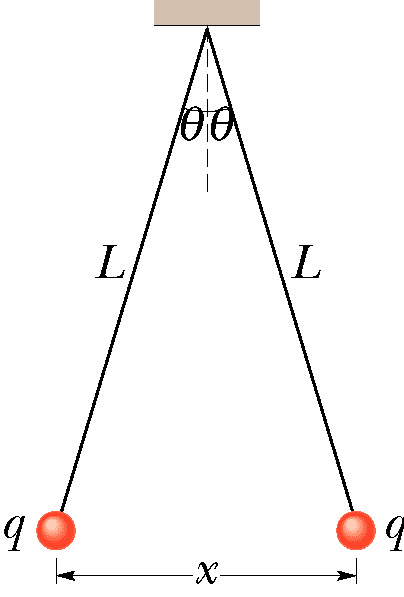# Two conducting balls hanging from the same point

rlc

## Homework StatementIn the figure, two conducting balls of identical mass m = 20 g and identical charge q hang from nonconducting threads of length L = 100 cm. If x = 5.9 cm, what is q? Since x is much smaller than L approximate sin(θ) by θ.

## Homework Equations

1. Tcos theta = mg
Tsin theta = ((kq^2)/x^2)
(x/(2L) = (kq^2)/(mgx^2))

## The Attempt at a Solution

Tcos theta = mg
Tsin theta = ((kq^2)/x^2)
Tan theta = sin theta/cos theta
solve for tangent
(x/(2L) = (kq^2)/(mgx^2))
then solve for q

This is what a classmate of mine posted as a way to solve the problem, but it hasn't been working for me. Does this look like the right method? What does the T before cos and sin mean?
Is it necessary to calculate theta, by sin(theta)=(0.059m/2)/(1 m) ....theta=1.69 deg ?

Homework Helper
Three forces work on each of the balls. If you draw them for one of the two you see what your classmate is doing.
You recognize mg and I suppose you also recognize ##kq^2\over x^2##. The balss can only hang still if the threads compensate both of these at the same time. T stands for tension: a thread pulls in a direction along the thread. That's all it can do! Grab one and play with it to confirm.

By the way, in math and in physics angles are measured in radians. For ##0<\alpha<\pi/2## you have ##\sin\alpha < \alpha<\tan\alpha## and for small ##\alpha## one can show ##\sin\alpha \approx\alpha\approx\tan\alpha## (try it on a calulator with radians)

Last edited:
rlc
Is the tension force something I can calculate from the problem?

Homework Helper
Is the tension force something I can calculate from the problem?
Well, if you wrote Tcos theta = mg you have already answered that !

rlc
Oh, so for that equation, I should solve for T. Then use that T in the next equation, leaving q as the only variable!
I'll fix my numbers for theta to make them radians.

Homework Helper
(x/(2L) = (kq^2)/(mgx^2))
then solve for q

Classmate passes ! No need for T, no need for theta.

•rlc
rlc
Question about SI units: do I need to convert x and L into meters, mass into kg?

Homework Helper
Is very good practice. For x/2L you can check that m/m gives the same result as cm/cm. You'll understand why, I hope ?

But the dimension of k forces you to use kg and m to get Coulomb.

ke = 8.9875517873681764×109 N·m2/C2

1 N = 1 kg m/s2

•rlc
rlc
(x/2L) = (kq^2)/(mgx^2)
(5.9 cm/(2*100 cm) = (9E9)q^2/( (0.020 kg)(9.81 m/s)(0.059 m)^2)
0.0295=(1.3178E13)q^2
2.2386E-15=q^2
q=4.73E-8 C

Does this look right to you? I'm on my last chance to input an answer :(

rlc
I just tried it and it worked!
Thank you so much for helping me. I really appreciate it.

Homework Helper
Good work!

I'm confused. we say tan theta = x/2L, or opposite/adjacent = x/2L. It makes sense that the opposite side is x/2, but that would leave the adjacent side as L, but we've already said the hypotenuse was L.

Am I messing something up?

Homework Helper
Hello Iason,Pretty old thread you are now resuming! Why not start a new one ?
To answer your question: You are not messing up at all, but if you are entitled to use ##\theta## for ##\sin\theta##, then you are also entitled to use ##\theta## for ##\tan\theta## (##\cos\theta=1##).

So: what you say is correct, but for small angles it's OK. (You could check -- not by solving the trigonometric equation, but by comparing ##\arctan(0.059)## with ##\arcsin(0.059)##.

And compare the situation if ##\theta = {\pi\over 4}##Hello Iason,And compare the situation if ##\theta = {\pi\over 4}##